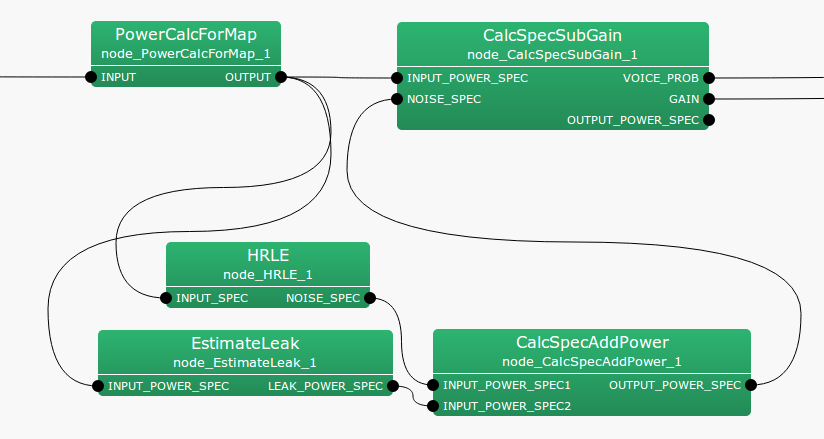### 6.3.4.1 Outline of the node

This node outputs the sum of two input spectra.

### 6.3.4.2 Necessary files

No files are required.

### 6.3.4.3 Usage

When to use

This node is used for noise estimation with the HRLE node. The power spectrum of noise estimated with the HRLE node is added to that of EstimateLeak so as to obtain a total noise power spectrum.

Typical connection

Figure 6.60 shows a connection example of CalcSpecAddPower . Inputs are the power spectrum of the noise estimated from HRLE and EstimateLeak . The output is connected with CalcSpecSubGain .Figure 6.60: Connection example of CalcSpecAddPower

### 6.3.4.4 Input-output and property of the node

Input

INPUT_POWER_SPEC1

: Map<int, ObjectRef> type. Vector<float> type data pair of a sound source ID and power spectrum.

INPUT_POWER_SPEC2

: Map<int, ObjectRef> type. Vector<float> type data pair of a sound source ID and a power spectrum.

Output

OUTPUT_POWER_SPEC

: Map<int, ObjectRef> type. Vector<float> type data pair of the sound source ID and the power spectra for which the two inputs are added.

Parameter

No prameters.

### 6.3.4.5 Details of the node

This node outputs the sum of two spectra.